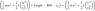# Work done dynamics question

• Hiccup
And if it's upward, is it still moving upward when it has dropped 1.5 m ? And what is the acceleration of the mass, you think ?In summary, The conversation discusses a physics homework problem involving an 8kg mass suspended from a grooved drum at radius r1=200mm and with a speed of 0.3m/s. The outer radius of the drum is r2=300mm and it has a radius of gyration k=210mm and mass 12kg. The question asks to determine the speed once the mass has dropped 1.5m. The conversation includes various attempts at solving the problem and discussing the use of equations such as s=r x theta and v=wr. The correct

#### Hiccup

Dear All, would really appreciate some help with the following question - am struggling with dynamics at the minute!
1. Homework Statement

An 8kg mass is suspended from a grooved drum at radius r1=200mm and with a speed of 0.3m/s it drops 1.5m.
The outer radius of the drum is r2=300mm. Assume that the drum has radius of gyration k=210mm and mass 12kg and a constant frictional torque in its bearings of 3Nm. Determine the speed once it has dropped the 1.5m.

## Homework Equations

So in a hint I have been told to utilise s=r x theta and also v=wr

## The Attempt at a Solution

I attempted the following:
mgh + 1/2m(mass)v^2 + 1/2 Iw(drum)^2 = 1/2m(mass)v^2 + 1/2 Iw(drum)^2

With the left hand side being the initial velocities with the 0.3m/s.

This threw me well out (the answer is supposed to calculate to 3.01m/s.

Anyone able to help, it would greatly appreciated!

Thanks!

Sorry, forgot to add - I had a -3 in the left hand side as the friction...

Well, did you work out your attempt ? How did you wrangle your -3 into the left hand side ? Is the friction there only initially ?

Hi BvU,
I was using the theory that,

T1 + ∑U1-2 = T2

Hence the 3Nm frictional torque was placed as a minus from the mgh on the left hand side. The frictional torque is stated as constant in the question hence I understand that it has to included as part the energy equation.
I did this problem earlier in the day and I believe I got something like 2 x10^-4 (!) so you can see I was well out...
I'm going to try it again as possibly tonight or tomorrow morning as I am flats out with assignments and revision at the moment so will stick up the answer I get the 2nd time around - never kn ow, I might get it right!

So to be a bit clearer on this and as sort of an answer to BvU, the following is the equation I have come up with to solve:
However, I cannot solve as I cannot work out the linear acceleration to calculate M; (M = F*s and the F is calculated from the tension in the rope which is T=m(g-a).
This as well as the unknown V^2 I (which is what I'm looking for) is really acting as a 'show stopper' for me.
Anyone??

Sorry, it won't let me post up the equation that I have copied and pasted from word!

#### Attachments

•2.4 KB · Views: 539
Why don't you type out a list of the numerical values you used in the equation?

m(block)=8kg
g=9.81m/s^2
r1=0.2m
r2=0.3m
k=0.21m
m(drum)-12kg
Torque(tf)=3Nm
u=0.3 m/s

I=mk^2 was used to calculate I of the drum
Theta was calculated from = s/r

 I started this yesterday but apparently forgot to post, sorry.

Although it is tempting to consider the dimension of a torque to be equal to the dimension of an energy, this is not the case: a torque is a (pseudo) vector and an energy is a scalar. So you can't just subtract the 3 from Δmgh.

Could you post your calculations in detail ? It enables more helpful assistance.

 Well, you did some of that, so that deserves a bit more help:

I suppose the initial 1/2 mu2 gave you 0.36 J ? That's what I get.
And the initial 1/2 I u2/r22 another 0.265 J ?

As I said, the ##\tau_f## can't just be added to ##mgh##, which is a substantial 118 J . Strange you should get such low final speed !

And what is ##M\theta## ? Same story as ##\tau_f## ? Didn't ##M\theta## change into ##{1\over 2} I\; \omega^2 \ ##?

Now comes the bummer: (since you dropped the supposed answer) I can't reconstruct the 3 m/s from the energies (0.5 J + 118 J - Wf =? about 60 J from 3 m/s).

Perhaps it's necessary to find the actual acceleration of the mass and use v = at with t from v0+at2 ?

θHi BvU, thanks for the input.
The question was handed in a tutorial on work done from uni with the answer given as 3.01m/s and I have since found this question in a Meriam textbook with the same answer but no worked example or one that is anything like it to draw from.

I included the Mθ as I assumed this was a rotational energy, so yes , same as the torque - this is incorrect I take it?

Rotational energy is already accounted for in ##{1\over 2} I\; \omega^2 ##, so this way you count it twice !

Any ideas how to deal with the friction already ?

And: -- just to be sure -- is the mass initially moving upward at 0.3 m/s or is it moving downward ?

Last edited: